# AP Chemistry : Concentration and Units

## Example Questions

← Previous 1

### Example Question #1 : Concentration And Units

A 100mL solution is composed of 25% ethanol by volume and water. What is the mass of the solution?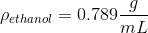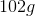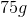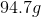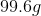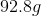Explanation:

First we determine the mass of the ethanol in solution using its density. Using the percent by volume of ethanol, we know that there are 25mL of ethanol in a 100mL solution. The remaining 75mL are water.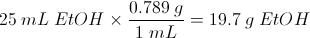Since the density of water is 1g/mL, we know that the mass of 75mL of water is 75g. The total mass is the sum of the ethanol and the water.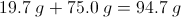### Example Question #2 : Identifying Unknown Concentration

What is the concentration if 40 g NaOH is dissolved in 1000 g of water

1 M
40 M
4 M
4 m
1 m
Explanation:

first of all, M = molar; m = molal- M = mol solute/ L of solution; m = mol solute/ kg solvent

you have 40 g NaOH * 1 mol/40 g = 1 mol

1000 g of water is equivalent to 1 L

1 mol/L = 1M

### Example Question #3 : Identifying Unknown Concentration

80.0g NaOH is put into 50000 mL water.  What is the molarity of the resulting solution?

0.00004 M

0.0016 M

0.04 M

0.625 M

1.6 M

0.04 M

Explanation:

Molarity = mol solute / L soution

mol solute = 80 g NaOH * 1 mol / 40 g = 2 mol

L solution = 50000 mL water * 1 L/1000 mL = 50 L

2 mol / 50 L =

### Example Question #1 : Identifying Unknown Concentration

How many liters of 10M HCl are needed to make 4L of 0.4M solution?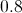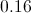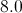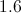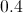Explanation:

A simple calculation can be done to perform any solution dilution problem. We know our equation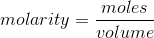.

We can rewrite this as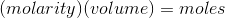.

Using this formula, we take the old solution and set it equal to the new solution.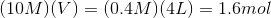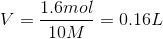We need 0.16 liters of our 10 molar solution.

### Example Question #1 : Titrations

Which solution has the highest molarity?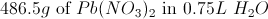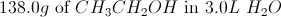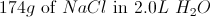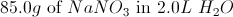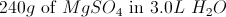Explanation:

This question requires us to calculate molarity for each answer choice. It is important to add everything correctly and be careful with more complex compounds.

Molarity is simply moles of solute over liters of solution. The correct answer, after trying each, is the answer with lead (II) nitrate, as it gets us a molarity of 2.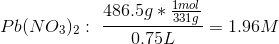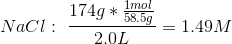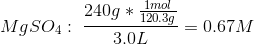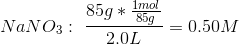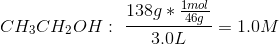### Example Question #21 : Solutions

Which of the following is equivalent to molarity?

Moles of solute per liter of solution

Kilograms of solute per mole of solution

Moles of solute per kilogram of solvent

Moles of solute per liter of solvent

Moles of solute per liter of solution

Explanation:

Molarity, molality, and normality are the three principle ways to measure concentration. Molarity is a measure of moles of solute per liter of solution. Molality is a measure of moles of solute per kilogram of solvent. Normality expressly relates to acids and bases, and is the measure of moles of solute divided by the number of hydrogen equivalents per mole, all divided by liters of solution. Normality is also referred to as "equivalents (of acid) per liter."

### Example Question #22 : Solutions

Given that 50 mL of 3M HI was present, what volume would be needed to change the concentration to 0.75M?

50mL

200mL

180mL

150mL

150mL

Explanation:

Use the equation M1V1 = M2V2, and plug in the corresponding values to solve for V2:

V2 = (3)(50)/(0.75) = 200mL

We started out 50mL of the solution, so you have to add 150mL to get a final volume of 200mL.

### Example Question #1 : Concentration And Units

What is the concentration of Ca in a solution of 1 mol CaCl2 in 1 L of distilled water? (M = molarity, m= molality)

2 m
1 m
1 M
Cannot be determined
2 M
Explanation:

The definition of molality is moles of solute in 1 kg of the solvent, whereas molarity is the number of moles of solute per 1 L of solutioin. Since 1 mol of CaCl2 is added to 1 L of water, this means that the volume of the final solution is greater than 1 L. Thus, molality is the more accurate concentration determinant, since the solution is probably close to 1 L.

### Example Question #6 : Identifying Unknown Concentration

Which of the following aqueous solutions is the most concentrated?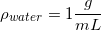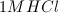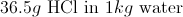All of these solutions have the same concentration.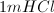Explanation:

In order to answer this question, it helps to know that 1 kilogram of water is equal to 1 liter of water, due to its density. Two of the above options refer to a 1m solution of hydrochloric acid. The other is a 1M solution.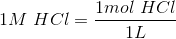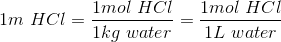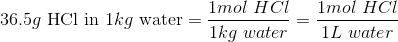All three of the options have the same amount of hydrochloric acid (one mole). For molarity, the hydrochloric acid is diluted with water until one liter of solution is created. For molality, one mole of HCl is added to one kilogram of water. Since one kilogram of water is one liter, this becomes the same concentration.

One a very small level, the 1M HCl solution will be slightly more concentrated. Creating a molal solution does not take into account the volume of the solute. If, for example, 100 cubic centimeters of HCl were added to one kilogram of water, the resulting volume would be more than one liter, making the concentration slightly less than 1M. This discrepancy is usually not accounted for in basic chemistry, but you should be familiar with the concept.

### Example Question #1 : Titrations

What is the molality of a solution created by mixing 4.3g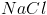into 43g of water?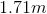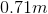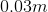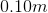Cannot be determinedExplanation:

Molality can be defined: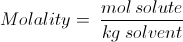It is slightly different from Molarity and has different uses.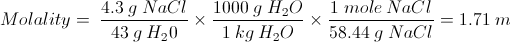← Previous 1